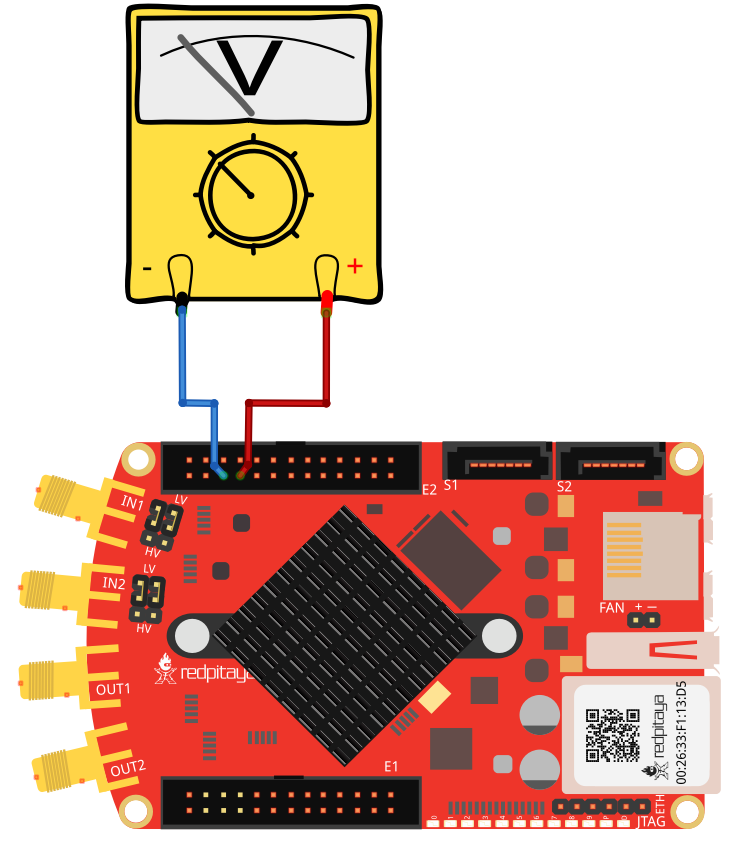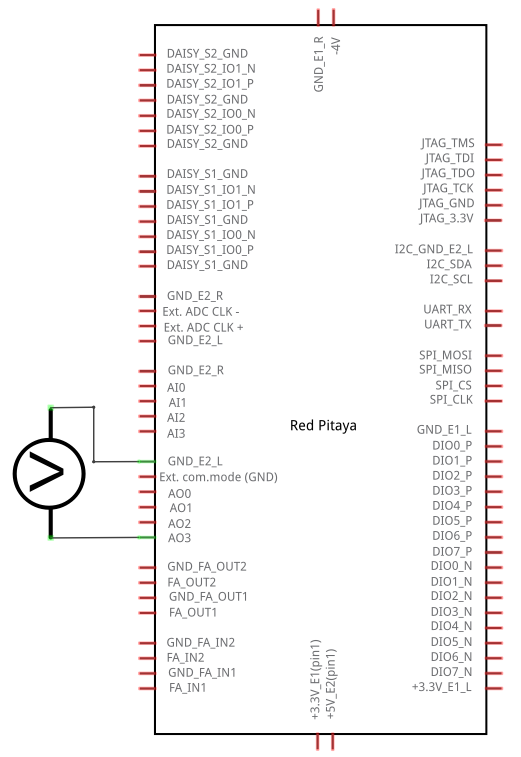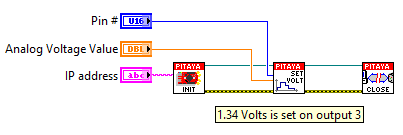# 2.3.1.4.2.2. Set analog voltage on slow analog output¶

## 2.3.1.4.2.2.1. Description¶

This example shows how to set analog voltage of slow analog outputs on Red Pitaya extension connector. Slow analog outputs on Red Pitaya are in range from 0 to 1.8 Volts.

## 2.3.1.4.2.2.2. Required hardware¶

• Red Pitaya device

• Voltmeter

Wiring example for STEMlab 125-14 & STEMlab 125-10:## 2.3.1.4.2.2.3. Circuit¶## 2.3.1.4.2.2.4. Code - MATLAB®¶

The code is written in MATLAB. In the code we use SCPI commands and TCP/IP communication. Copy code from below to MATLAB editor, save project and press run.

```%% Define Red Pitaya as TCP/IP object
IP= '192.168.178.108';          % Input IP of your Red Pitaya...
port = 5000;
tcpipObj=tcpip(IP, port);

%% Open connection with your Red Pitaya

fopen(tcpipObj);
tcpipObj.Terminator = 'CR/LF';

fprintf(tcpipObj,'ANALOG:PIN AOUT0,0.3');  % 0.3 Volts is set on output 0
fprintf(tcpipObj,'ANALOG:PIN AOUT1,0.9');
fprintf(tcpipObj,'ANALOG:PIN AOUT2,1');
fprintf(tcpipObj,'ANALOG:PIN AOUT3,1.5');

fclose(tcpipObj);
view rawanalog_outputs.m
```

## 2.3.1.4.2.2.5. Code - C¶

Note

C code examples don’t require the use of the SCPI server, we have included them here to demonstrate how the same functionality can be achieved with different programming languages. Instructions on how to compile the code are here -> link

```/* Set analog voltage on slow analog output */

#include <stdio.h>
#include <stdlib.h>

#include "rp.h"

int main (int argc, char **argv) {
float value ;

// Voltages can be provided as an argument (default is 1V)
for (int i=0; i<4; i++) {
if (argc > (1+i)) {
value [i] = atof(argv[1+i]);
} else {
value [i] = 1.0;
}
printf("Voltage setting for AO[%i] = %1.1fV\n", i, value [i]);
}

// Initialization of API
if (rp_Init() != RP_OK) {
fprintf(stderr, "Red Pitaya API init failed!\n");
return EXIT_FAILURE;
}

// Setting a voltage for each ananlog output
for (int i=0; i<4; i++) {
int status = rp_AOpinSetValue(i, value[i]);
if (status != RP_OK) {
printf("Could not set AO[%i] voltage.\n", i);
}
}

// wait for user input
getchar();

// Releasing resources
rp_Release();

return EXIT_SUCCESS;
}
```

## 2.3.1.4.2.2.6. Code - Python¶

```#!/usr/bin/python

import sys
import redpitaya_scpi as scpi

rp_s = scpi.scpi(sys.argv)

value = [1,1,1,1]
for i in range(4):
if len(sys.argv) > (i+2):
value[i] = sys.argv[i+2]
print ("Voltage setting for AO["+str(i)+"] = "+str(value[i])+"V")

for i in range(4):
rp_s.tx_txt('ANALOG:PIN AOUT' + str(i) + ',' + str(value[i]))
```

## 2.3.1.4.2.2.7. Code - LabVIEW¶